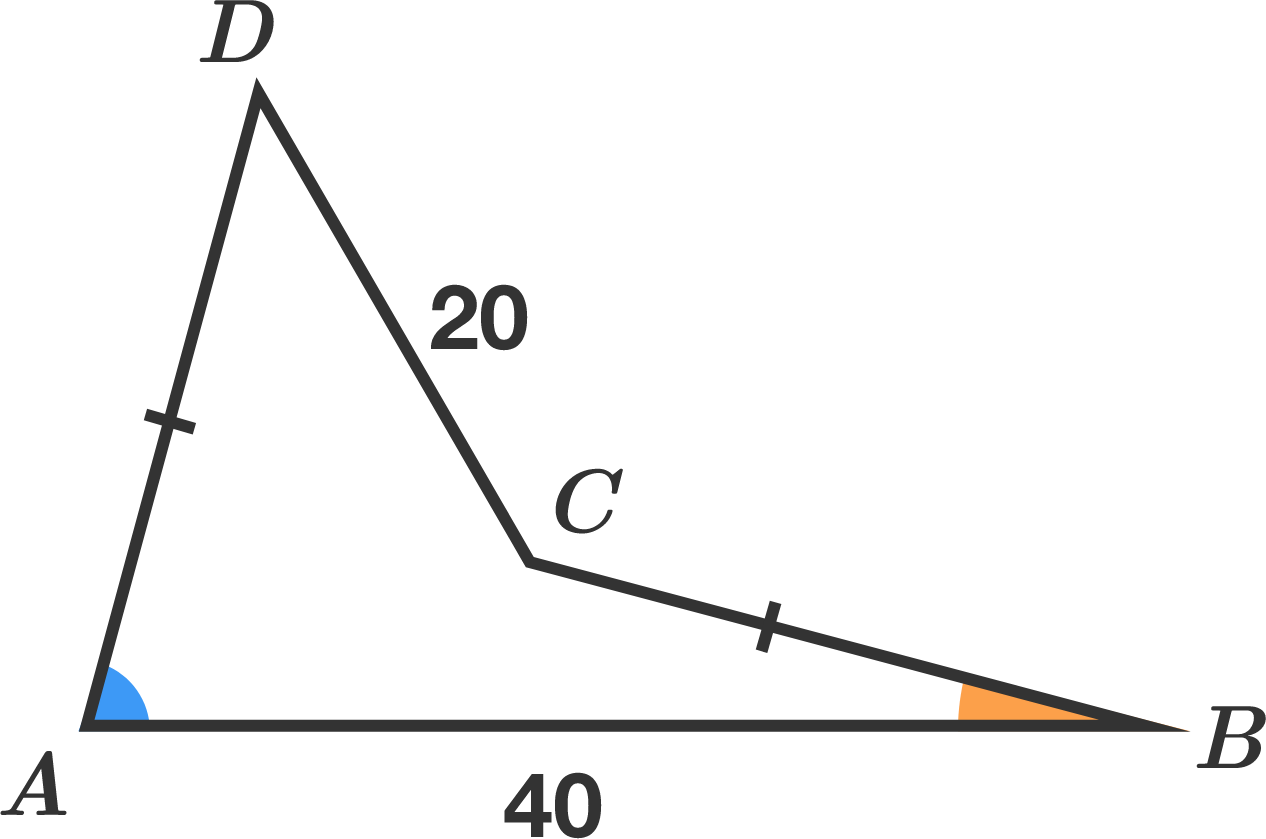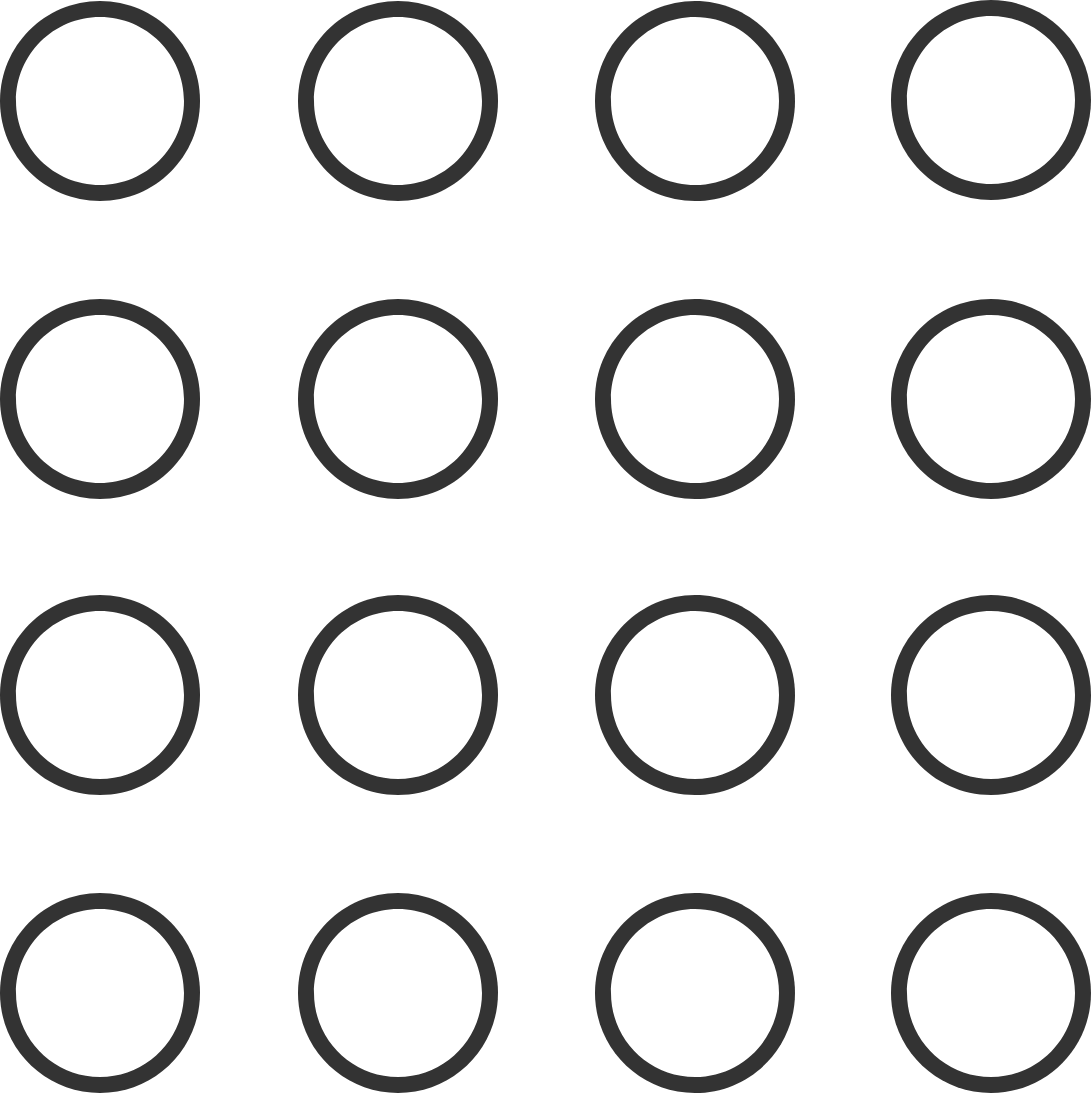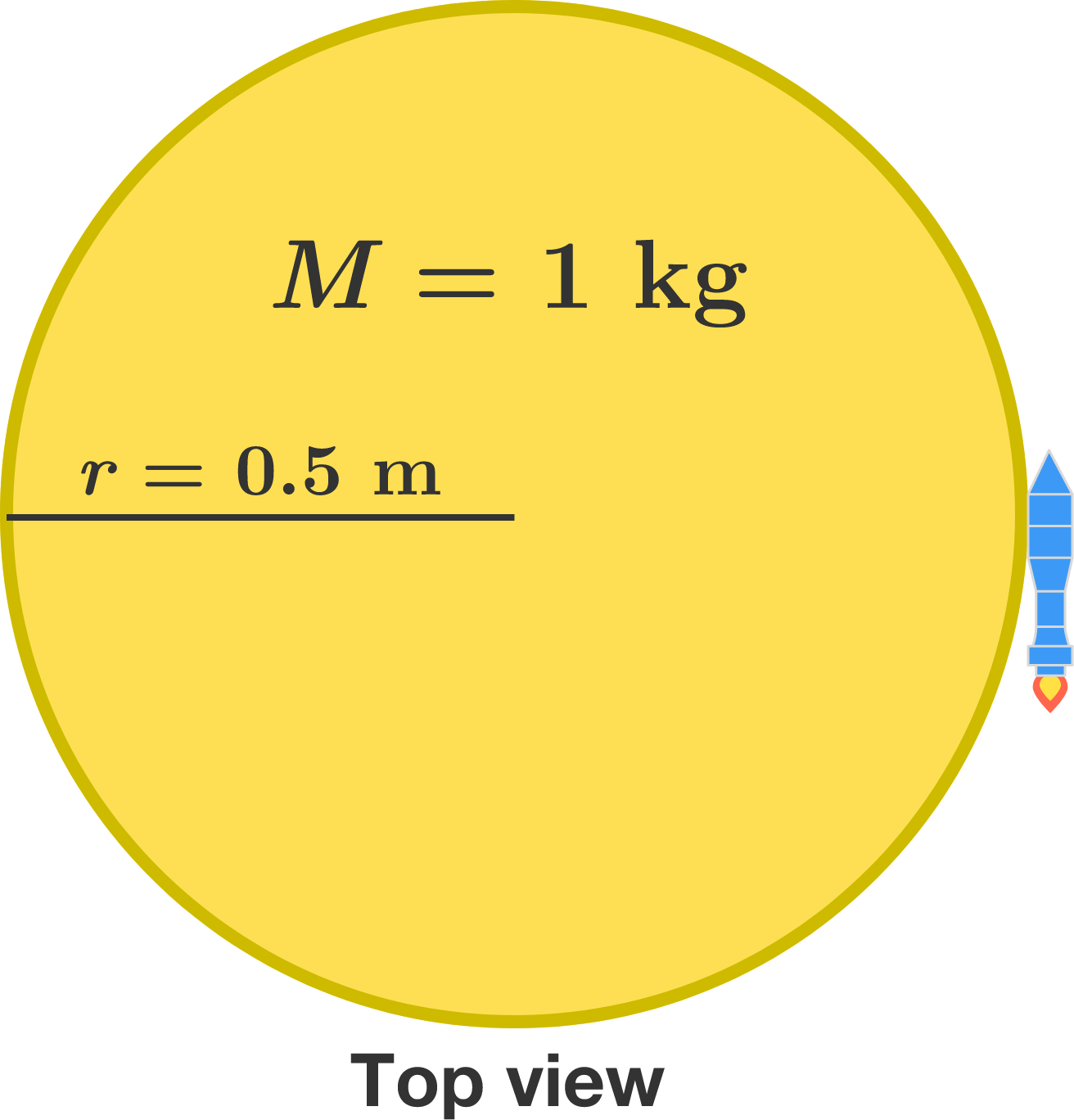# Problems of the Week

Contribute a problem

$ABCD$ is a quadrilateral with $AD=BC,$ $AB=40,$ $CD=20,$ and ${\color{#3D99F6}m\angle A} + {\color{#EC7300}m\angle B} = 90^\circ.$

What is the area of quadrilateral $ABCD?$Hint: Consider how copies of $ABCD$ can be constructed into another shape.

Let $x, y,$ and $z$ be real numbers satisfying $\dfrac{x}{y+z}+\dfrac{y}{z+x}+\dfrac{z}{x+y}=1.$

Find the maximum value of $\dfrac{x^2}{y+z}+\dfrac{y^2}{z+x}+\dfrac{z^2}{x+y}.$Tom is jumping around a $4\times4$ grid of circles with the following conditions:

• He starts from any of the 16 circles.
• He always jumps in a straight line from one circle to any other circle.
• Each subsequent jump is farther than the last.
• He doesn't visit a circle already visited.

The maximum number of circles that he can step on is $m,$ and the number of ways of doing so is $r.$

Find the value of $mr.$


Note: The grid is fixed on the ground, so a method obtained by rotating another is considered different.

$128=2^7,$ but none of the other permutations of the digits of 128 form powers of 2: $182,\ 218,\ 281,\ 812,\ 821.$

Is there any power of 2, $2^n,$ such that at least one of its other permutations is also a power of 2?A uniform disk sits on a smooth floor with a rocket strapped to its perimeter. One second after the rocket ignites, how far is the disk's center from where it started, in meters?


Details and Assumptions:

• The mass of the disk is $M=\SI{1}{\kilo\gram},$ its radius is $r=\SI{0.5}{\meter},$ and the rocket provides a constant thrust of $T = \SI{10}{\newton}$ after it ignites.
• Neglect the mass and size of the rocket.